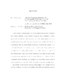## Adaptive Constraint Reduction for Convex Quadratic Programming and Training Support Vector Machines##### Files
umi-umd-5144.pdf(2.39 MB)
Convex quadratic programming (CQP) is an optimization problem of minimizing a convex quadratic objective function subject to linear constraints. We propose an adaptive constraint reduction primal-dual interior-point algorithm for convex quadratic programming with many more constraints than variables. We reduce the computational effort by assembling the normal equation matrix with a subset of the constraints. Instead of the exact matrix, we compute an approximate matrix for a well chosen index set which includes indices of constraints that seem to be most critical. Starting with a large portion of the constraints, our proposed scheme excludes more unnecessary constraints at later iterations. We provide proofs for the global convergence and the quadratic local convergence rate of an affine scaling variant. A similar approach can be applied to Mehrotra's predictor-corrector type algorithms. An example of CQP arises in training a linear support vector machine (SVM), which is a popular tool for pattern recognition. The difficulty in training a support vector machine (SVM) lies in the typically vast number of patterns used for the training process. In this work, we propose an adaptive constraint reduction primal-dual interior-point method for training the linear SVM with $l_1$ hinge loss. We reduce the computational effort by assembling the normal equation matrix with a subset of well-chosen patterns. Starting with a large portion of the patterns, our proposed scheme excludes more and more unnecessary patterns as the iteration proceeds. We extend our approach to training nonlinear SVMs through Gram matrix approximation methods. Promising numerical results are reported.